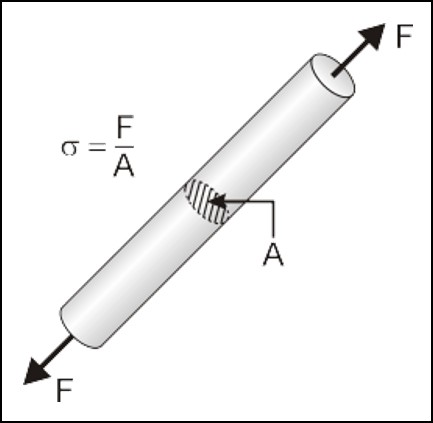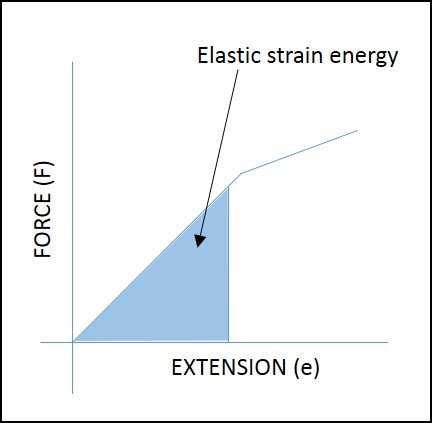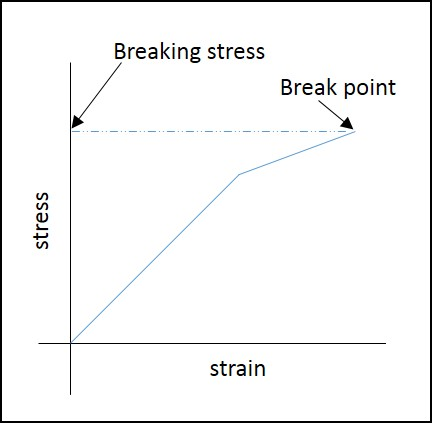# Stress & Strain

Introduction

Ever wondered why, when iron rod can withstand more load, things made of glass or wood breaks easily? The answer lies in behaviour of materials to external loading. This article discusses some of the important properties of the materials that are used to study the effect of when an external load is applied to materials.

A. Tensile Stress

When a spring or a wire is stretched, tensile force is applied to it. Tensile force per unit area of a cross-section is called tensile stress, σ. The SI units of tensile stress are N m^-2.How to Calculate Tensile Stress

Tensile stress can be calculated from experimental data. Consider an iron rod with cross sectional area (A) of 2 m^2. Tensile force (F) of 500 N is applied on both sides of the rod as shown in Figure 1.Figure 1: Tensile Stress CalculationB. Tensile Strain

When a spring or a wire is stretched, tensile force is applied to it. Extension per unit length of the material is called tensile strain (ε). Tensile strain has no units since it is a ratio of two lengths.How to Calculate Tensile Strain

Tensile strain is calculated from experimental data. Consider a 2 m-long iron rod. Tensile force acting on the rod elongates it by 0.02 m, as shown in Figure 2.Figure 2: Tensile Strain CalculationC. Elastic Strain Energy

Within elastic limit, when an object has its shape changed due to force acting on it, the object can do work as it returns to its original shape when the force is removed. Energy is stored in the form of potential energy when it is strained. This form of energy is called elastic strain energy.How to Calculate Elastic Strain Energy

Elastic strain energy can be calculated from a graph of force (F) against extension (e). Elastic strain energy is represented by the area under the line as shown in Figure 3.Figure 3: Elastic strain energy

D. Breaking Stress

Breaking stress of a material is the tensile stress at which the material breaks. It is also called the tensile strength of the material. Figure 4 shows the breaking stress on a stress against strain graph.Figure 4: Breaking Stress

E. Plastic Deformation

Beyond the elastic limit, materials do not obey Hooke’s law. Any deformation beyond that point will be permanent. This deformation beyond the elastic limit is known as plastic deformation. Proportionality limit (P) and Elastic limit (E) is illustrated in the below stress vs. strain graph.Figure 5: Elastic Limit

F. Brittle Failure

When a material fails with little or no deformation, then it is called brittle failure. On the other hand, materials that can sustain deformation before breaking exhibit ductile failure.

G. Applications of Stress and Strain:

Stress, strain and plastic deformation properties are used in the design of machine components such as gears, chains, and machine columns.

H. Summary

Tensile stress, tensile strain, strain energy, breaking stress, elastic and plastic deformations and brittle failure are important properties of a material. These properties are widely used in designing machine components.

• Tensile force per unit area of cross-section is called tensile stress, σ.
• Extension per unit length of the material is called tensile strain, ε.
• Elastic strain energy is energy stored in a body due to change of shape.
• The breaking stress of a material is the tensile stress at which the material breaks.
• Any deformation beyond the elastic limit is called plastic deformation.
• Metal failure with little or no deformation is called brittle failure.